# Anisotropy analysis

The Minkowski tensors contain information about both the preferred direction and the amplitude of the anisotropy. The latter can be conveniently extracted by scalar anisotropy indices.

Here, we present some common indices in both 2D and 3D.

## Irreducible Minkowski Tensors (IMTs)

They provide the most systematic, sensitive and convenient characterization of interfacial anisotropy in a two-phase medium.
In particular, they characterize anisotropy w.r.t. different symmetries.

They are presented in detail in the main article: Anisotropy analysis by IMT

## Ratio of Eigenvalues

The Cartesian representation of the second-rank Minkowski tensors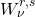(with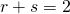) allows for a straightforward definition of anisotropy indices w.r.t. different geometrical properties (both translation invariant and covariant):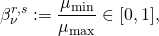where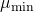and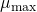are the smallest and largest eigenvalues of.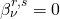indicates a “flat” body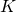.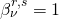indicates an “isotropic” body, in the sense that it has a statistically identical mass distribution in any set of three orthogonal directions; this includes the sphere, but also regular polyhedra and the FCC, BCC and HCP Voronoi cells.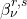has be successfully applied to a variety of simulated and experimental systems.

In 2D, the index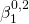of the surface tensor is equivalent to the IMT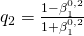.

## Isoperimetric Ratio

A truly classical index of anisotropy is the isoperimetric ratio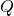.

In 2D: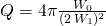In 3D: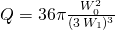In this sense, it is the dimensionless quotient of the volume and surface of an object. It is normalized so that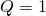for a ball.

Different shape information is contained in the isoperimetric ratio and the Minkowski anisotropy indices, as can be seen in the comparison ofandfor various geometric shapes: